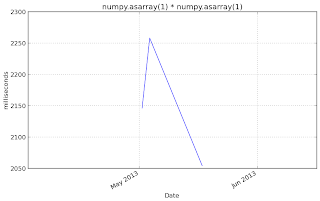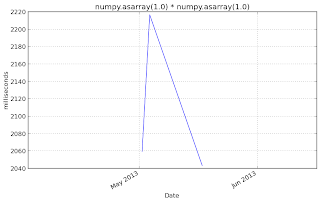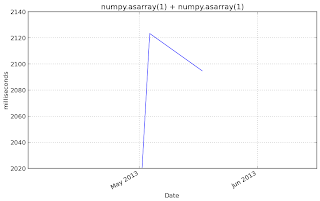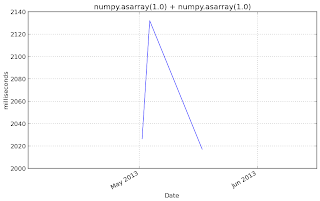## Friday, May 17, 2013

### Performance parity between numpy arrays and Python scalars

Small numpy arrays are very similar to Python scalars but numpy incurs a fair amount of extra overhead for simple operations. For large arrays this doesn't matter, but for code that manipulates a lot of small pieces of data, it can be a serious bottleneck.

### For example:

```
In : x = 1.0

In : numpy_x = np.asarray(x)

In : timeit x + x
10000000 loops, best of 3: 61 ns per loop

In : timeit numpy_x + numpy_x
1000000 loops, best of 3: 1.66 us per loop
```

I tried to introduced, a short path (at present) for integer and float addition of numpy array. In umath/ufunc_type_resolution.c , ufunc lookup loop find best data types based on input operands types. In short path, rather than going to loop again for addition operation, it return the best known data type.
```
/* Short path for addition of int + int */
int key = 0;
for (j = 0; j < nargs; ++j) {
key = (key<<5) + dtypes[j]->type_num;
}
NPY_UF_DBG_PRINT1("key is %d\n",key);

int rent = -1;
if(key == 7399)
rent = 7;
else if(key == 12684)
rent = 13;

NPY_UF_DBG_PRINT1("rent is %d\n",rent);
if(rent > 0){
*out_innerloop = ufunc->functions[rent];
*out_innerloopdata = ufunc->data[rent];
NPY_UF_DBG_PRINT1("type @ hashposition %d\n",rent);
return 0;
}
}
```

### Following are the benchmark result based on vbench for numpy

All operations have been run 1000000, with timeit function. And results for commits after 2013, May, 1 have been taken.

#### Multiplication

timeit.timeit('x * x',setup='import numpy as np;x = np.asarray(1)')numpy.asarray(1) * numpy.asarray(1)

timeit.timeit('x * x',setup='import numpy as np;x = np.asarray(1.0)')numpy.asarray(1.0) * numpy.asarray(1.0)

timeit.timeit('x * y',setup='import numpy as np;x = np.asarray(1.0);y = np.asarray(1)')numpy.asarray(1) * numpy.asarray(1.0)

timeit.timeit('x + x',setup='import numpy as np;x = np.asarray(1)')numpy.asarray(1) + numpy.asarray(1)

`timeit.timeit('x + y',setup='import numpy as np;x = np.asarray(1.0)';y = np.asarray(1)')`numpy.asarray(1) + numpy.asarray(1.0)

`timeit.timeit('x + x',setup='import numpy as np;x = np.asarray(1.0)')`numpy.asarray(1.0) + numpy.asarray(1.0)

Benchmark for numpy can be found on github